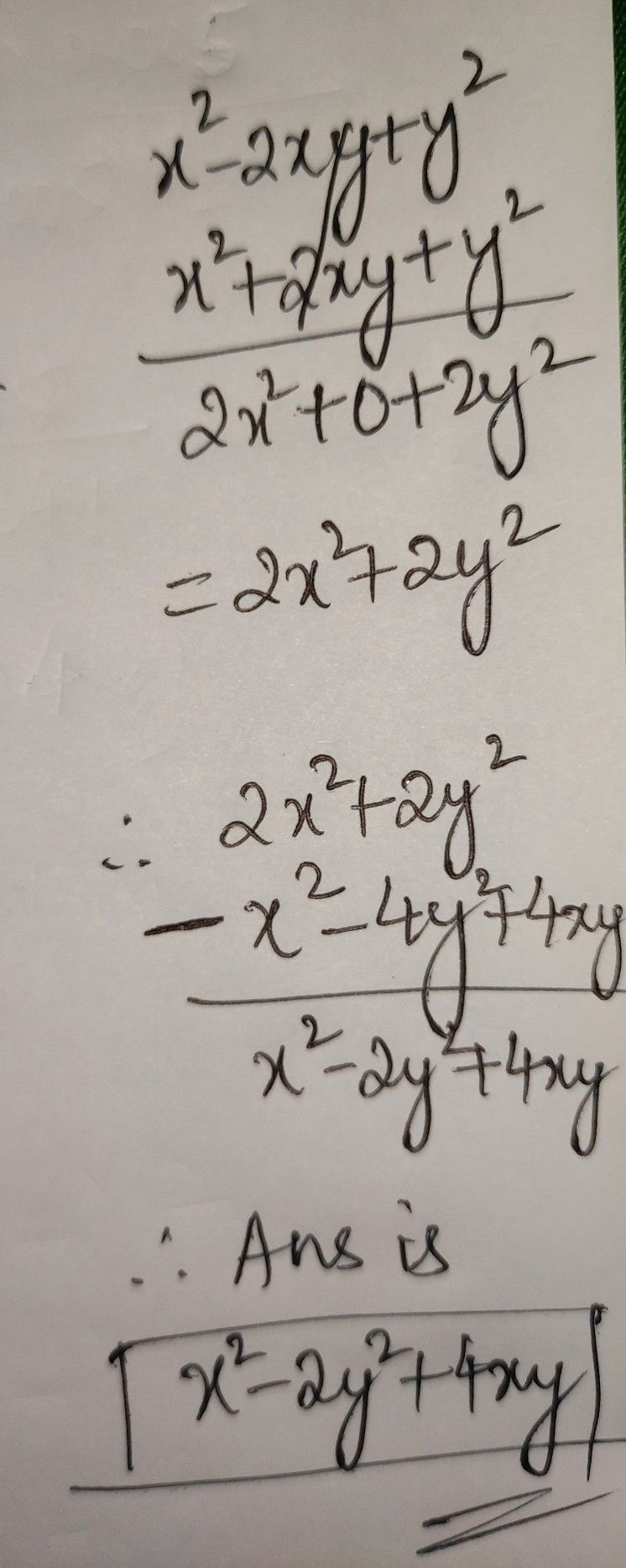Symbol
ProblemSubtract $tx^{2}-4xy+4y^{2}$ from the sum of $x^{2}$ $-2xy+y^{2}$ and $x^{2+2xy+y^{2}}$